## Example Questions

### Example Question #1 : How To Find The Degree Of A Polynomial

Find the degree of the polynomial: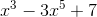Explanation:

To find the degree of a polynomial we must find the largest exponent in the function.

The degree of the polynomialis 5, as the largest exponent ofis 5 in the second term.

### Example Question #2 : How To Find The Degree Of A Polynomial

What is the degree of the polynomial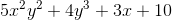?Explanation:

When a polynomial has more than one variable, we need to find the degree by adding the exponents of each variable in each term.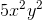has a degree of 4 (since both exponents add up to 4), so the polynomial has a degree of 4 as this term has the highest degree.

### Example Question #3 : How To Find The Degree Of A Polynomial

Find the degree of the following polynomial: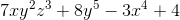Explanation:

When a polynomial has more than one variable, we need to find the degree by adding the exponents of each variable in each term.

Even though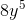has a degree of 5, it is not the highest degree in the polynomial -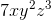has a degree of 6 (with exponents 1, 2, and 3). Therefore, the degree of the polynomial is 6.

### Example Question #4 : How To Find The Degree Of A Polynomial

Solve each problem and decide which is the best of the choices given.

What is the degree of the following polynomial?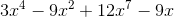Explanation:

The degree is defined as the largest exponent in the polynomial. In this case, it is.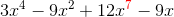### Example Question #5 : How To Find The Degree Of A Polynomial

What is the degree of this polynomial?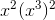Degree 6

Degree 10

Degree 12

Degree 8

Degree 7

Degree 8

Explanation:When an exponent with a power is raised to another power, the value of the power are multiplied.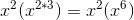When multiplying exponents you add the powers together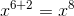The degree of a polynomial is the determined by the highest power. In this problem the highest power is 8.

### Example Question #1 : How To Find The Degree Of A Polynomial

Find the degree of the following polynomial: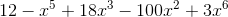Here, the term with the largest exponent is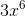, so the degree of the whole polynomial is 6.